### Type to search# Python Basics : Learning Python by Simple Real World Problems : Problem#1

##### Share

Prerequisites To Follow this Exercise :

1. Python Installed in your System : If you are a new user to Python and want to know how to install Python via the Anaconda Distribution , You can go through the step by step Blog i have written to install Python via Anaconda Distribution & start using Jupyter Notebook : https://instrovate.com/2019/06/09/python-anaconda-distribution-how-to-download-and-install-it-and-run-the-first-python-program/

Once you have the  Python Installed in your system you are Good to Go ahead and follow the below Use Case and Example.

Problem Statement :

Priyanka and Brooklyn are two python programmers. Priyanka writes more lines of code than Brooklyn per day. Brooklyn being very enthusiastic person decided to improve on herself. Currently, Brooklyn is ‘D’ problems behind Priyanka. So, Brooklyn increased her speed to writing ‘K’ lines of code per day. Priyanka continue to write her code at ‘A’ lines of code per day.

Write a python program that accepts three arguments i.e. D, K and A. and returns the number of days Brooklyn will take to bypass Priyanka.

Solution & Explanation:

Suppose Priyanka have written 200 lines of code and Brooklyn have written 150 lines of codes. Current speed of Priyanka is 20 lines per day and now Brooklyn has increased her speed to 25 lines per day.

So, based on this following are our inputs:

D = 200 – 150 = 50

K = 25

A = 20

So, Brooklyn has to cover 50 lines of code in total. Each day Brooklyn can cover the difference in speed with Priyanka i.e. each day Brooklyn can cover (25-20) i.e. 5 lines of code per day.

Since she has to cover 50 lines, so total number of days required to surpass Priyanka will be:

( D / (K – A) ) + 1

The point here must be noted that if the value of A > K this means speed of Priyanka is still greater than that of Brooklyn then Brooklyn will not be able to surpass Priyanka. Mathematically it will result in negative answer. SO, while writing the code we should put a check that value of K entered should be greater than value of A in order to get correct outcome.

Program

We will be using Anaconda Python distribution version 2.7 and Spyder IDE to write the python program. You can install python and download Anaconda from the Anaconda website

Once you open Spyder , it would look like below :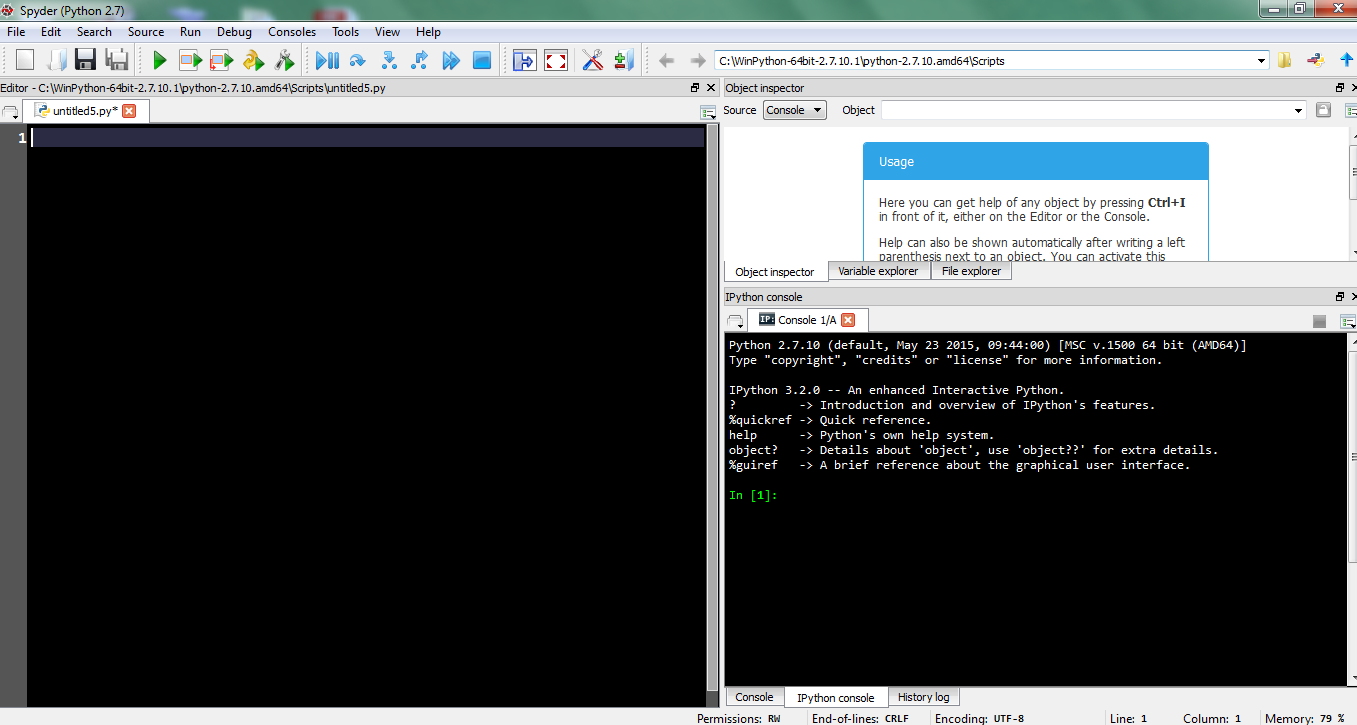The first step is to take input from user for the three values D, A and K. The user can be made to enter

Input from python by using input function. The text in the quotes can be written in the input function which will be displayed before user is prompted to enter the input. The input text entered by user is accepted in python as a string. So, we also need to convert it into an integer type. Below is the screenshot to do it: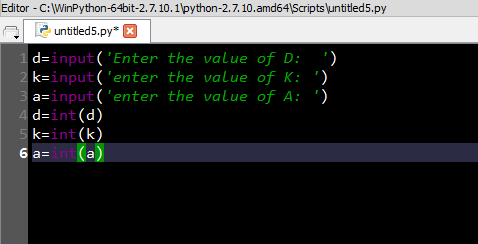Next is to check the whether the value of K is greater than A or not. So, if the value of K is less than A then more computation is possible and program should exit. So, to exit the program we need to import the library sys. And use the sys.exit() function to quit the program. This can be done programmatically as follows: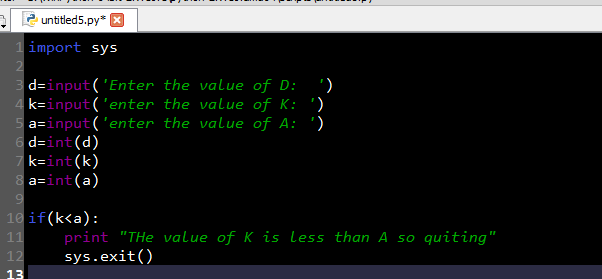Now since we have checked the constraint we can proceed to compute the desired outcome. The code can be written as follows: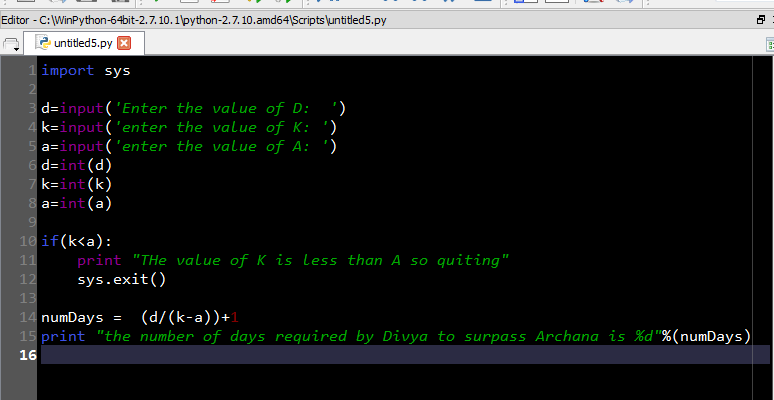Now we will run the program. IN Spyder python can be executed the clicking the green arrow at the icon bar as follows: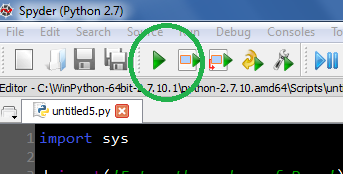By clicking this following is the execution outcome of the code: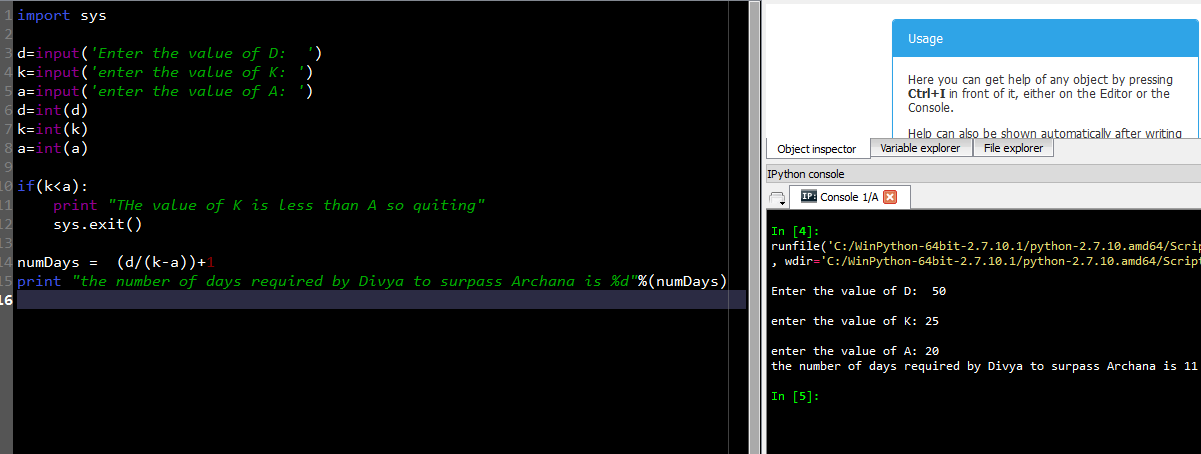Hence the answer is 11 Days as can be seen is the above screenshot.

For Corporate Training contact info@instrovate.com

Tags: